# CBSE Class 10 Physics Reflection Refraction Of Light Worksheet

Read and download free pdf of CBSE Class 10 Physics Reflection Refraction Of Light Worksheet. Students and teachers of Class 10 Science can get free printable Worksheets for Class 10 Science Chapter 10 Light Reflection and Refraction in PDF format prepared as per the latest syllabus and examination pattern in your schools. Class 10 students should practice questions and answers given here for Science in Class 10 which will help them to improve your knowledge of all important chapters and its topics. Students should also download free pdf of Class 10 Science Worksheets prepared by school teachers as per the latest NCERT, CBSE, KVS books and syllabus issued this academic year and solve important problems with solutions on daily basis to get more score in school exams and tests

## Worksheet for Class 10 Science Chapter 10 Light Reflection and Refraction

Class 10 Science students should refer to the following printable worksheet in Pdf for Chapter 10 Light Reflection and Refraction in Class 10. This test paper with questions and answers for Class 10 will be very useful for exams and help you to score good marks

### Class 10 Science Worksheet for Chapter 10 Light Reflection and Refraction

MULTIPLE CHOICE QUESTIONS

Question : Rays from sun converge at a point 15 cm in front of a concave mirror. Where should an object be placed so that size of its image is equal to the size of the object?
(a) 15 cm in front of the mirror
(b) 30 cm in front of the mirror
(c) between 15 cm and 30 cm in front of the mirror
(d) more than 30 cm in front of the mirror

Question : To determine the focal length of a concave mirror by forming image of a distant object, the screen should be placed
(a) in any direction
(b) inclined at angle of 45°
(c) at right angle to the plane of mirror
(d) parallel to the plane of mirror

Question : You are given water, mustard oil, glycerine and kerosene. In which of these media, a ray of light incident obliquely at same angle would bend the most?
(a) Kerosene
(b) Water
(c) Mustard oil
(d) Glycerine

Question : How will the image formed by a convex lens be affected if the upper half of the lens is wrapped with a black paper?
(a) The size of the image is reduced to one-half.
(b) The upper half of the image will be absent.
(c) The brightness of the image is reduced.
(d) There will be no effect

Question : Beams of light are incident through the holes A and B and emerge out of box through the holes C and D respectively, as shown in the figure. Which of the following could be inside the box?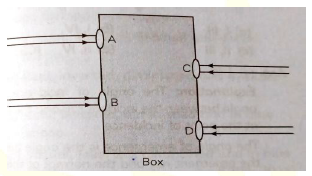(a) A rectangular glass slab
(b) A convex lens
(c) A concave less
(d) A prism

Question : When an object is kept within the focus of a concave mirror, an enlarged image is formed behind the mirror. This image is:
(a) real
(b) inverted
(c) virtual and inverted
(d) virtual and erect

Question : A full length image of a distant tall building can definitely be seen by using:
(a) a concave mirror
(b) a convex mirror
(c) a plane mirror
(d) both concave as well as plane mirror

Question : Magnification produced by a rear view mirror fitted in vehicles:
(a) is less than one
(b) is more than one
(c) is equal to one
(d) can be more than or less than one depending upon the position of the object in front of it.

Question : A student determines the focal length of a device 'X' by focusing the image of a distant object on a screen placed 20 cm from the device on the same side as the object. The device 'X' is
(a) Concave lens of focal length 10 cm
(b) Convex lens of focal length 20 cm
(c) Concave mirror of focal length 10 cm
(d) Concave mirror of focal length 20 cm

Question : A small bulb is placed at the focal point of a converging lens. When the bulb is switched on, the lens produces:
(a) a convergent beam of light
(b) a divergent beam of light
(c) a parallel beam of light
(d) a patch of coloured light

ASSERTION AND REASON

Directions: In the following questions, a statement of assertion (A) is followed by a statement of reason (R). Mark the correct choice as:
(a) If both assertion and reason are true and reason is the correct explanation of assertion
(b) If both assertion and reason are true but reason is not the correct explanation of assertion
(c) If assertion is true but reason is false.
(d) If both assertion and reason are false.

Question : Assertion: A ray incident along normal to the mirror retraces its path.
Reason: In reflection, angle of incidence is always equal to angle of reflection.

Question : Assertion: Higher is the refractive index of the medium, lesser is the velocity of light in that medium.
Reason: Refractive index of a medium is inversely proportional to the velocity of light.

Question : Assertion: When a concave mirror is held in water, its focal length will decrease.
Reason: The focal length of a concave mirror depends on the density the medium in which it is placed.

Question : Assertion: A convex lens is made of two different materials. A point object is placed on the principal axis. The number of images formed by the lens will be two.
Reason : The image formed by convex lens is always virtual.

Question : Assertion: Full length image of a distant object, such as a tall building, can be seen in a convex mirror.
Reason: A convex mirror has a greater focal length than a concave mirror of the same aperture.

CASE BASED QUESTIONS

Light is a form of energy which induces sensation of vision to our eyes. It becomes visible when it bounces off on surfaces and hits our eyes. The phenomenon of bouncing back of light rays in the same medium on striking a smooth surface is called reflection of light.
If parallel beam of incident rays remains parallel even after reflection and goes only in one direction is known as regular reflection. It takes place mostly in plane mirrors
or highly polished metal surfaces. The mirror outside the driver side of a vehicle is usually a spherical mirror and printed on such a mirror is usually the warning "vehicles in this mirror are closer than they appear."

Question : Which type of mirror is used outside the driver's side of a vehicle?
(a) Plane mirror
(b) Concave mirror
(c) Convex mirror
(d) Magic mirror

Question : No matter how far you stand from a mirror, your image appears erect. The mirror can be
(a) Plane
(b) Concave
(c) convex
(d) Either plane or convex

Question : Which of the following diagrams represents the image formation in above case?(a) (i)
(b) (ii)
(c ) (iii)
(d) (iv)

Question : If an object is placed at 10 cm from a convex mirror of radius of curvature 60 cm,then find the position of image.
(a) 4 cm
(b) 7.5 cm
(c) 10 cm
(d) 12.5 cm

Question : The focal length of mirror is 12 cm. The radius of curvature is
(a) 12 cm
(b) 24 cm
(c) 20 cm
(d) 36 cm

2. The refractive index of a medium with respect to vacuum is called absolute refractive index of the medium. It is given by, μ = sin i/sinr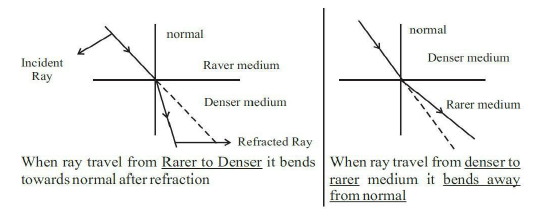Absolute refractive indices of some of the materials A, B, C and D are given in the following table :
Medium    Refractive Index
A              1.54
B              1.33
C              2.42
D              1.65

Question : In which of the materials given in the above table, light travels fastest?
(a) A
(b) B
(c) C
(d) D

Question : The speed of light in air is 3x108 ms-1 and that in medium A is 2.5 x 10 ms-1. The refractive index of A will be
(a) 1.2
(b) 0.5
(c) 4.5
(d) 1.5

Question : When light travels from air to glass,
(a) angle of incidence > angle of refraction
(b) angle of incidence < angle of refraction
(c) angle of incidence = angle of refraction
(d) Can't say

Question : The refractive index of P with respect to Qis 2. Find the refractive index of Q with respect to P.
(a) 0.5
(b) 0.2
(c) 2
(d) 2.5

Question : A student determines the focal length of a device X, by focusing the image of a far off object on the screen positioned as shown in the figure below: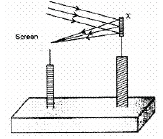The device X is a :
a. Convex lens
b. Concave mirror
c. Concave lens
d. Convex lens

Explanation: Because the screen is on the same side of the object which means it is never a lens becoz it happens behind the lenses in such case.
Moreover Concave mirror forms real images i.e. image can be obtained on a screen.

Question :  Which of the following diagrams give a correct picture?Explanation: All rays passing parallel to the axis will pass through the focus after refraction in the lens.

Question :  When light enters from air to glass, which of the following changes:
A. Wavelength
B. Velocity
C. Frequency
D. Amplitude
a. A and D
b. B and C
c. A and C
d. A, B and D

Explanation: When light enters from one medium into another, the frequency of light does not change. Speed, wavelength and amplitude may change depending upon the optical density of the two mediums.

Question :  If f1 and f2 are the focal lengths of two lenses, what is the relation for equivalent focal length?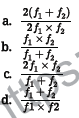Question : If the angle of incidence is , what is the angle of refraction?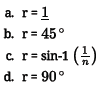Question : While doing experiment with candle to find focal length of a concave mirror, the candle is placed between:
a. pole and focus
b. at focus
c. focus and centre of curvature
d. beyond focus

Explanation: For objects between f and 2f or centre of curvature the image formed will be enlarged and beyond C.

Question :  Match the following with the correct response:
(1) Plane mirror           (A) Virtual image, inverted and large
(2) Concave lens          (B) Virtual image, erect and same size
(3) Convex lens           (C) Virtual image, positive focal length
(4) Convex mirror        (D) Real image, negative focal length, negative power
a. 1-B, 2-D, 3-A, 4-C
b. 1-C, 2-B, 3-D, 4-A
c. 1-A, 2-C, 3-B, 4-D
d. 1-D, 2-A, 3-C, 4-B

Explanation: The virtual image formed by a plane mirror is virtual, erect and of the same size as that of the object. According to New Cartesian Sign Convention, the focal length (and power) of a convex lens is positive, and that of a concave lens is negative. A concave lens always produces erect and virtual images. A convex mirror always forms virtual images that are erect and diminished. The focal length of a convex mirror is positive, and that of a concave mirror is negative. A convex lens produces real and enlarged images when the object is placed beyond the centre of curvature.

### Light Reflection And Refraction Assertion Reason Class 10 Science

A. Both assertion and reason are true, and reason is the correct explanation of assertion.
B. Both assertion and reason are true, but reason is not the correct explanation of assertion.
C. Assertion is true but reason is false.
D. Assertion is false and reason is true.

Question. Assertion (A): A Concave mirror of radius R is placed in water .Its focal length differs in Air and Water.
Reason (R): Focal length of concave mirror is equal to R/2.

Question. Assertion (A): The emergent ray is parallel to the direction of incident ray.
Reason (R): The extent of bending of the ray of light at the opposite parallel faces (air- glass interface and glass- air interface) of the rectangular glass slab is equal and opposite.

Question. Assertion(A) : For observing traffic at back, the driver mirror is convex mirror.
Reason (R) : A convex mirror has much larger field of view than a plane mirror.

Question. Assertion(A) : When the object moves with a velocity 2 m/s, its image in the plane mirror moves with a velocity of 4 m/s.
Reason (R) : The image formed by a plane mirror is as far behind the mirror as the object is in front of it.

Question. Assertion (A): Refractive index of glass with respect to air is different for red light and violet light.
Reason (R): Refractive Index of a pair of medium does not depend on the wavelength of the light used.

Question. Assertion (A): Higher the Refractive index of the medium lesser will be the speed of light in that Medium.
Reason (R): Refractive index is inversely proportional to the speed of light.

Question. Assertion(A) : The formula connecting u, v and f for a spherical mirror is valid in all situations for all spherical mirrors for all positions of the object.
Reason (R) : Laws of reflection are strictly valid for plane surfaces.

Question. Assertion(A): The mirrors used in search lights are concave spherical.
Reason (R) : In concave spherical mirror the image formed is always virtual.

Question : Name a point inside a lens such that a ray of light passing through it goes undeviated.

Question : What is magnification of lens?
Answer : Magnification of a lens is to the ratio of the height of the image formed by lens to the actual height of object.
If h is the height of the object and h' the height of the image formed by lens, then magnification
m = h'/h
If u and v are the distances of object and image
m = h'/h = v/u

Question : What kind of image can be obtained on the screen?

Question : Name the type of mirror which always forms a virtual and diminished image.

Question : Give the cartesian sign convention for:
a. height of a real image, and
b. height of a virtual image.
Answer :  (a) - ve, and (b) + ve.

Question : Name the kind of surfaces that
i. Reflect
ii. Refract most of the light falling on them.
Answer : i. Polished opaque surfaces.
ii. Transparent surfaces.

Question. State the laws of refraction of light. Explain the term absolute refractive index of a medium’ and write an expression to relate it with the speed of light in vacuum.
Answer: (a) Laws of refraction of light:
(i) The incident ray, the refracted ray and the normal to the interface of two transparent media at the point of incidence, all lie in the same plane.
(ii) The ratio of sine of angle of incidence to the sine of the angle of refraction is constant, for the light of a given colour and for the given pair of media This law is also known as Snell’s law of refraction.
sini/sinr = constant,
where i is the angle of incidence and r is the angle of refraction.
This constant value is called refractive index of the second medium with respect to the first when the light travels from first medium to second medium.
⇒ constant = n21 = v1/v2 ∴ sini/sinr = v1/v2
If n is the absolute refractive index of the medium, c is the velocity of light in vacuum and v is the speed of light in a given medium, then n = c/v.

Question. Draw ray diagrams to show the formation of three times magnified (a) real, and (b) virtual image of an object by a converging lens. Mark the positions of O, F and 2F in each diagram.
Answer: (b) Ray diagrams of an object placed between F1 and optical centre O of lens can be drawn as follows:
(i) The image formed is virtual and erect.
(ii) Image is formed in front of the lens.
(iii) Image formed is enlarged.

Question. If the image formed by a spherical mirror for all positions of the object placed in front of it is always erect and diminished, what type of mirror is it? Draw a labelled ray diagram to support your answer.
Answer: If the image formed by a spherical mirror is always erect and diminished then it is convex mirror.

Question. The linear magnification produced by a spherical mirror is -1. Analysing this value state the (i) type of mirror and (ii) position of the object with respect to the pole of the mirror. Draw any diagram to justify your answer.
Answer: (i) Concave mirror because the image is real, inverted.
(ii) Object is placed at C.

Question. State the two laws of reflection of light.
Answer: Laws of reflection of light states that
(i) The angle of incidence is equal to the angle of reflection.
(ii) The incident ray, the reflected ray and the normal to the mirror at the point of incidence all lie in the same plane.

Question : A mirror has magnification 0.4, what type of the mirror is that? and what type of the image is formed ?
Answer :  It is a convex mirror since the magnification is positive as well as less than one. Image is diminished and erect

Question :  Find the position, nature and size of the image formed by a convex lens of focal length 12 cm of an object 5 cm high placed at a distance 20 cm from it.Question : State the laws of reflection of light.
Answer : Laws of reflection of light are:
(i) The angle of incidence is equal to the angle of reflection and
(ii) The incident ray, the normal to the mirror at the point of incidence and the reflected ray, all lie in the same plane.

Question : Which is optically denser out of the two medium M1 = 1.71 (refractive index) and M2 = 1.36 (refractive index). How does speed of light change when it travels from optically rarer to denser medium.
Answer : Medium M1 with refractive index 1.71 is optically denser than the other medium M2.
Speed of light decreases when it travels from rarer to denser medium.

Question : What are the properties of the image formed by plane mirror?
Answer : Image formed by plane mirror is always virtual and erect. The size of the image is equal to that of the object. The image formed is as far behind the mirror as the object is in front of it and the image is laterally inverted.

Question : What is refractive index?
Answer : If ‘i’ is the angle of incidence and ‘r’ is the angle of refraction then
sin i / sin r = Constant
This constant value is called the refractive index of the second medium with respect to the first.

Question : Give the uses of concave mirrors.
Answer : (i) Concave mirror are used in torches, search-lights and vehicle headlights to get powerful parallel beams of light.
(ii) They are also used as shaving mirrors to see a large image of the face.
(iii) Dentists use concave mirrors to see large images of the teeth of patients.
(iv) Large concave mirrors are used in making solar furnaces, solar cookers etc.

Question : What is absolute refractive index of the medium?
Answer : When the refractive index of medium 2 is considered with respect to vacuum. This is called the absolute refractive index of the medium.
2/n = Speed of light in 2 / Speed of light in 1

Question : Define power of lens. What is the S. I. unit of power of a lens? If power of lens is +2D what is the nature and focal length of the lens?
Answer : Power of lens: The degree of convergence or divergence of light rays obtained by a lens is expressed in terms of its power. Power of a lens is defined as the reciprocal of its focal length.
P = 1/f
S.I. unit of power of a lens is ‘diopter’
P = +2 D.
Lens is convex and the focal length of the lens is +0.50 m.
(P = 1/f, 2 D = 1/f, ∴ f = 1/2 = 0.05)

Question : When a ray of light entering from air is incident on the surface of a glass slab at an angle of 90°, what will be the measure of angle of refraction. Why does a ray change its path when it passes from one medium to another medium?
Answer : The angle of refraction will be zero. A light ray changes its path when it passes from one medium to another medium.

Question : Give the uses of convex mirrors.
Answer : Convex mirrors are commonly used as rear-view mirrors in vehicles. As these mirrors can give an erect image, wider field of view, these mirrors are used in vehicles to see the traffic behind.

Question : Refractive index of kerosene oil is 1.44 and that of water is 1.33. A ray of light enters from kerosene oil to water. Where would light ray bend and why?
Answer : A ray of light enters from kerosene oil to water i.e., refractive index 1.44 to 1.33 i.e., from denser to rarer medium. Hence the ray of light bends away from the normal.

Question : Define pole and centre of curvature of spherical mirrors.
Answer : Pole: The centre of the reflecting surface of a spherical mirror is a point called the pole. It lies on the surface of the mirror. It is represented by “P”. Centre of Curvature: The reflecting surface of a spherical mirror forms a part of a sphere this sphere has a centre and this point is called the centre of curvature of the spherical mirror.

Question : Comment on the size, position of the image formed by a concave mirror of focal length 18 cm when an object is placed:
(i) at 22 cm (ii) 14 cm (ii) 40 cm.
in front of mirror without calculations.
Answer : (i) When the object is placed at 22 cm, the image is formed beyond 36 cm, real, inverted image is magnified.
(ii) When the object is at 14 cm then the image formed is virtual, behind the mirror and magnified.
(iii) When the object is placed beyond 40 cm, then the image is formed between 18 cm and 36 cm, it is real inverted and diminished image.

Question : Give the laws of refraction of light.
Answer : The laws of refraction of light are
(i) The incident ray, the refracted ray and the normal to the interface of two transparent media at the point of incidence all lie in the same plane.
(ii) The ratio of sine of angle of incidence to the sine of angle of refraction is constant, for the light of a given colour and for the given pair of media. This law is also known as Snell’s law of refraction.

Question : A rod of length 10 cm lies along the principal axis of a concave mirror of 10 cm in such a way that the end closer to the pole is 20 cm away from it. Find the length of image?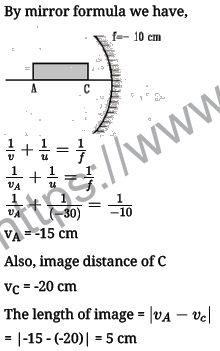Question : An object is placed at a distance of 10 cm from a convex mirror of focal length 15 cm. Find the position and nature of the image.
Answer : u = –10 cm [u is always negative]; f = 15 cm [ convex mirror] v = ?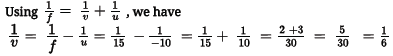So v = 6 cm behind the mirror or towards left of the mirror. Image is virtual and erect.

Question : Why do we prefer a convex mirror as a rear-view mirror in vehicles?
Answer : Convex mirrors are used as rear-view mirrors in vehicles to see the traffic at the rear side. Convex mirrors are preferred because
a. they always give an erect, though diminished image.
b. they have a wider field of view as they are curved outwards.
c. Thus, convex mirrors enables the driver to view much larger area than a plane mirror.

Question. (a) Water has refractive index 1.33 and alcohol has refractive index 1.36. Which of the two medium is optically denser? Give reason for your answer.
(b) Draw a ray diagram to show the path of a ray of light passing obliquely from water to alcohol.
(c) State the relationship between angle of incidence and angle of refraction in the above case.
Answer: (a) Here, alcohol is optically denser medium as its refractive index is higher than that of water. When we compare the two media, the one with larger refractive index is
called the optically denser medium than the other as the speed of light is lower in this medium.
(b) Since light is travelling from water (rarer medium) to alcohol (denser medium), it slows down and bends towards the normal.
where i = angle of incidence and r = angle of refraction.
(c) According to Snell’s law,
sini/sinr=μalcohol /μwater =1.36/1.33 = 1.0225
∴ sin i = 1.0225 × sin r

Question. What is meant by power of a lens? Write its SI unit. A student uses a lens of focal length 40 cm and another of -20 cm. Write the nature and power of each lens.
Answer: Power is the degree of convergence or divergence of light rays achieved by a lens.
It is defined as the reciprocal of its focal length.
i.e., P = 1/f
Given that: Focal length of lens A, fA = +40 cm
Focal length of lens B, fB = -20 cm
Lens A is converging. Lens B is diverging.

Question. A concave mirror has a focal length of 20 cm. At what distance from the mirror should a 4 cm tall object be placed so that it forms an image at a distance of 30 cm from the mirror? Also calculate the size of the image formed.
Answer: Given f = -20 cm v = -30 cm, u = ?
Using 1/v + 1/u = 1/f
1/u = 1/f – 1/v = 1/(−20) – /(−30) = (−3+2)/60
⇒ u = -60 cm
∴ Object placed at 60 cm from the mirror.
Also magnification, m = h′/h = −v/u
⇒ h’ = −(−30)/−60 × 4 = -2 cm
∴ The size of the image is 2 cm.

Question. The image of an object formed by a mirror is real, inverted and is of magnification -1.
If the image is at a distance of 40 cm from the mirror, where is the object placed?
Where would the image be if the object is moved 20 cm towards the mirror? State reason and also draw ray diagram for the new position of the object to justify your answer.
Answer: Since the image formed by the mirror is real and inverted, therefore the mirror is concave and magnification of the mirror will be
m = –v/u ⇒ -1 = –v/u ⇒ v = u
i.e., object and image both are formed at the centre of curvature, i.e., 40 cm from the mirror.
Now, if the object is moved 20 cm towards the mirror, the object will be at the focus of the mirror and therefore the image will be formed at infinity.

Question : i. Draw a ray diagram to show the formation of image by a convex lens when an object is placed in front of the lens between its optical centre and principal focus.
ii. In the above ray diagram mark the object distance (u) and the image distance (v) with their proper signs (+ ve or - ve as per the new cartesian sign convention) and state how these distances are related to the focal length (f) of the convex lens in this case.
iii. Find the power of convex lens which forms a real and inverted image of magnification -1 of an object placed at a distance of 20 cm from its optical centre.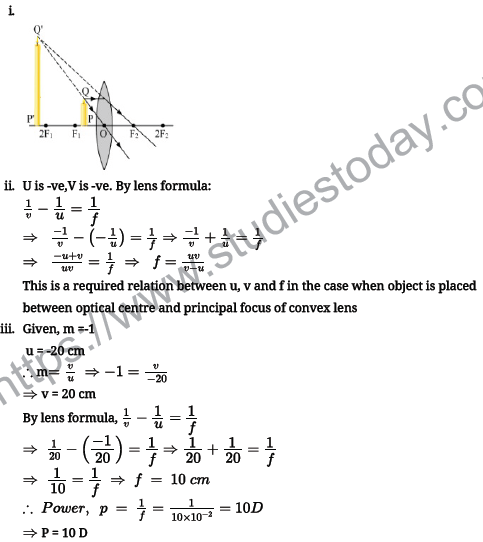Question : How are the images formed in convex mirror when object is moved from infinity to the mirror?
Answer :  Object at Infinity: Rays from infinity come parallel to principal axis. Ray AB is reflected towards BH and ray EG is reflected towards GJ. When produced back they meet at F, the principal focus (Rule 1).
An extremely small, erect, virtual image is formed at F.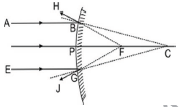Object at infinity: A virtual, erect, extremely diminished image is formed at F.
Object placed anywhere except infinity. A ray AD parallel to principal axis after reflection appears to pass through F. Another ray AE normal to mirror and appearing to pass through C is reflected back along the same path. They appear to meet at A' forming image of A and A'. Similarly, image of B is formed at B'. This virtual, erect image of AB is formed at A'B' between P and F behind the mirror. Image is erect and diminished in size.Object any where, A virtual, erect, diminished image is formed behind the mirror between F and P

Question : How can you show that if a ray enters a rectangular glass slab obliquely and emerges from the opposite face, the emergent ray is parallel to the incident ray.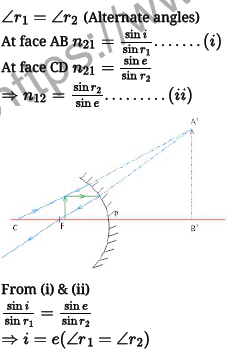Question : It is desired to obtain an erect image of an object, using concave mirror of focal length of 12 cm.

i. What should be the range of distance of an object placed in front of the mirror?
ii. Will the image be smaller or larger than the object? Draw ray diagram to show the formation of image in this case.
iii. Where will the image of this object be, if it is placed 24 cm in front of the mirror?
Draw ray diagram for this situation also to justify your answer. Show the positions of pole, principal focus and the centre of curvature in the above ray diagrams.
i. In concave mirror erect image is formed only when the object is placed between pole and focus. Thus, range to obtain erect image is 0 < u < 12.
ii. The image would be larger than the objectiii. If the object distance is 24 cm which is the approx position of centre of curvature, then the image will be formed at the same position. Here, f = -12 cm, u =-24 cm, v=?
By using mirror formula,Question. (i) A doctor has prescribed a corrective lens of power +1.5 D. Find the focal length of the lens. Is the prescribed lens diverging or converging?
(ii) A concave lens of focal length 15 cm forms an image 10 cm from the lens. How far is the object placed from the lens? Draw the ray diagram.
Answer: (i) Power of lens (P) = 1/f
P = 1.5D
f = 1/1.5 = 10/15 = 0.66 m
A convex lens has a positive focal length. Therefore, it is a convex lens or a converging lens.
(ii) Focal length of concave lens (OF1), f = – 15 cm
Image distance, v= – 10 cm
According to the lens formula,
The negative value of u indicates that the object is placed 30 cm in front of the lens.
This is shown in the following ray diagram.

Question. Rishi went to a palmist to show his palm. The palmist used a special lens for this purpose.
(i) State the nature of the lens and reason for its use. (ii) Where should the palmist place/hold the lens so as to have a real and magnified image of an object?
(iii) If the focal length of this lens is 10 cm, the lens is held at a distance of 5 cm from the palm, use lens formula to find the position and size of the image.
Answer: (i) The lens used here is a convex lens and it is used as a magnifying glass because at close range, i.e., when the object is placed between optic centre and principal focus it forms an enlarged, virtual and erect image of the object.
(ii) When this lens is placed such that the object is between the centre of curvature and the principal focus, the palmist obtain a real and magnified image.
(iii) Given focal length, f = 10 cm and u = -5 cm According to lens formula, Thus, the image will be formed at 10 cm on the same side of the palm and the size of the image will be enlarged.

Question. (a) A 5 cm tall object is placed perpendicular to the principal axis of a convex lens of focal length 20 cm. The distance of the object from the lens is 30 cm. Find the position, nature and size of the image formed.
(b) Draw a labelled ray diagram showing object distance, image distance and focal length in the above case.
Answer: (a) Given, h = 5 cm, f = 20 cm, u = -30 cm
Using lens formula, 1/v – 1/u = 1/f
1/v=1/u+1/f=1/(−30)+1/20=(−2+3)/60=1/60
⇒ v = 60 cm
Now, magnification, m = h′/h = v/u
⇒ h’ = v/u × h = 60/(−30) × 5 = -10 cm
Hence, the image formed at 60 cm, which is real and magnified.

1. The refractive index of carbon disulphide is 1.63. What is the meaning of this statement?

2. A person wants to see the full length image of tall building in a small mirror. Which type of mirror should be used by him?

3. Distinguish between real and virtual images.

4. A ray of light travelling in air enters obliquely into water. Does the light ray bend towards or away from the normal? Why?

5. What is lateral displacement of light? State the factors affecting it

6. Name the type of mirror used in the following situations:

(i) Head light of a car

(ii) Rear-view mirror of vehicles

(iii) Solar furnace

7. Under what condition will a glass lens placed in a transparent liquid becomes invisible?

8. Draw a ray diagram and also state the nature of the image formed by concave mirror when the object is placed at the centre of curvature.

9. Draw a diagram to show dispersion of white light by a glass prism. Label the coloures that appear at the two ends of the colour band. State the reason why different coloured rays deviate differentially in the prism.

10. With a help of a ray diagram, state the meaning of refraction of light. State Snell’s law of refraction of light and also express it mathematically.

## More Study Material

### CBSE Class 10 Science Chapter 10 Light Reflection and Refraction Worksheet

We hope students liked the above worksheet for Chapter 10 Light Reflection and Refraction designed as per the latest syllabus for Class 10 Science released by CBSE. Students of Class 10 should download in Pdf format and practice the questions and solutions given in the above worksheet for Class 10 Science on a daily basis. All the latest worksheets with answers have been developed for Science by referring to the most important and regularly asked topics that the students should learn and practice to get better scores in their class tests and examinations. Studiestoday is the best portal for Class 10 students to get all the latest study material free of cost.

### Worksheet for Science CBSE Class 10 Chapter 10 Light Reflection and Refraction

Expert teachers of studiestoday have referred to the NCERT book for Class 10 Science to develop the Science Class 10 worksheet. If you download the practice worksheet for one chapter daily, you will get higher and better marks in Class 10 exams this year as you will have stronger concepts. Daily questions practice of Science worksheet and its study material will help students to have a stronger understanding of all concepts and also make them experts on all scoring topics. You can easily download and save all revision worksheet for Class 10 Science also from www.studiestoday.com without paying anything in Pdf format. After solving the questions given in the worksheet which have been developed as per the latest course books also refer to the NCERT solutions for Class 10 Science designed by our teachers

#### Chapter 10 Light Reflection and Refraction worksheet Science CBSE Class 10

All worksheets given above for Class 10 Science have been made as per the latest syllabus and books issued for the current academic year. The students of Class 10 can be rest assured that the answers have been also provided by our teachers for all worksheet of Science so that you are able to solve the questions and then compare your answers with the solutions provided by us. We have also provided a lot of MCQ questions for Class 10 Science in the worksheet so that you can solve questions relating to all topics given in each chapter. All study material for Class 10 Science students have been given on studiestoday.

#### Chapter 10 Light Reflection and Refraction CBSE Class 10 Science Worksheet

Regular worksheet practice helps to gain more practice in solving questions to obtain a more comprehensive understanding of Chapter 10 Light Reflection and Refraction concepts. Worksheets play an important role in developing an understanding of Chapter 10 Light Reflection and Refraction in CBSE Class 10. Students can download and save or print all the worksheets, printable assignments, and practice sheets of the above chapter in Class 10 Science in Pdf format from studiestoday. You can print or read them online on your computer or mobile or any other device. After solving these you should also refer to Class 10 Science MCQ Test for the same chapter.

#### Worksheet for CBSE Science Class 10 Chapter 10 Light Reflection and Refraction

CBSE Class 10 Science best textbooks have been used for writing the problems given in the above worksheet. If you have tests coming up then you should revise all concepts relating to Chapter 10 Light Reflection and Refraction and then take out a print of the above worksheet and attempt all problems. We have also provided a lot of other Worksheets for Class 10 Science which you can use to further make yourself better in Science

Where can I download latest CBSE Printable worksheets for Class 10 Science Chapter 10 Light Reflection and Refraction

You can download the CBSE Printable worksheets for Class 10 Science Chapter 10 Light Reflection and Refraction for latest session from StudiesToday.com

Can I download the Printable worksheets of Chapter 10 Light Reflection and Refraction Class 10 Science in Pdf

Yes, you can click on the links above and download Printable worksheets in PDFs for Chapter 10 Light Reflection and Refraction Class 10 for Science

Are the Class 10 Science Chapter 10 Light Reflection and Refraction Printable worksheets available for the latest session

Yes, the Printable worksheets issued for Class 10 Science Chapter 10 Light Reflection and Refraction have been made available here for latest academic session

How can I download the Class 10 Science Chapter 10 Light Reflection and Refraction Printable worksheets

You can easily access the links above and download the Class 10 Printable worksheets Science Chapter 10 Light Reflection and Refraction for each chapter

Is there any charge for the Printable worksheets for Class 10 Science Chapter 10 Light Reflection and Refraction

There is no charge for the Printable worksheets for Class 10 CBSE Science Chapter 10 Light Reflection and Refraction you can download everything free

How can I improve my scores by solving questions given in Printable worksheets in Class 10 Science Chapter 10 Light Reflection and Refraction

Regular revision of practice worksheets given on studiestoday for Class 10 subject Science Chapter 10 Light Reflection and Refraction can help you to score better marks in exams

Are there any websites that offer free test sheets for Class 10 Science Chapter 10 Light Reflection and Refraction

Yes, studiestoday.com provides all latest NCERT Chapter 10 Light Reflection and Refraction Class 10 Science test sheets with answers based on the latest books for the current academic session

Can test papers for Class 10 Science Chapter 10 Light Reflection and Refraction be accessed on mobile devices

Yes, studiestoday provides worksheets in Pdf for Chapter 10 Light Reflection and Refraction Class 10 Science in mobile-friendly format and can be accessed on smartphones and tablets.

Are worksheets for Chapter 10 Light Reflection and Refraction Class 10 Science available in multiple languages

Yes, worksheets for Chapter 10 Light Reflection and Refraction Class 10 Science are available in multiple languages, including English, Hindi